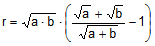Problem 279: Tangent Circles, Common External Tangent, Chords, Inradius. Level: High School, SAT Prep, College geometry.

In the figure below, the circles of centers A and B with radius a and b, respectively, are tangent externally at point C.
If DE is the common external tangent and r is the inradius of triangle DCE, prove that.Recent Additions